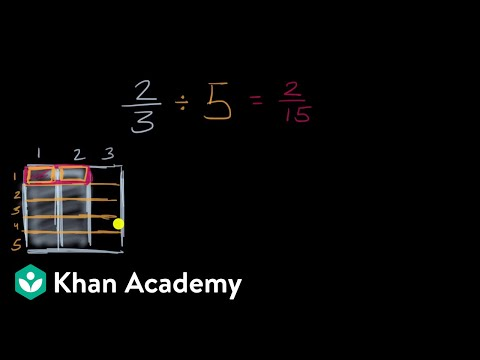Video

# Dividing fractions: 3/5 ÷ 1/2 (Full video)

Khan Academy

## Supporting grades: 6

Description: Dividing two fractions is the same as multiplying the first fraction by the reciprocal of the second fraction. The first step to dividing fractions is to find the reciprocal (reverse the numerator and denominator) of the second fraction. Next, multiply the two numerators. Then, multiply the two denominators. Finally, simplify the fractions if needed. Created by Sal Khan and Monterey Institute for Technology and Education.

You must log inorsign upif you want to:*

*Teacher Advisor is 100% free.

### Other videos you might be interested in### Dividing Fractions Word Problems (Full video)

#### Student Achievement Partners